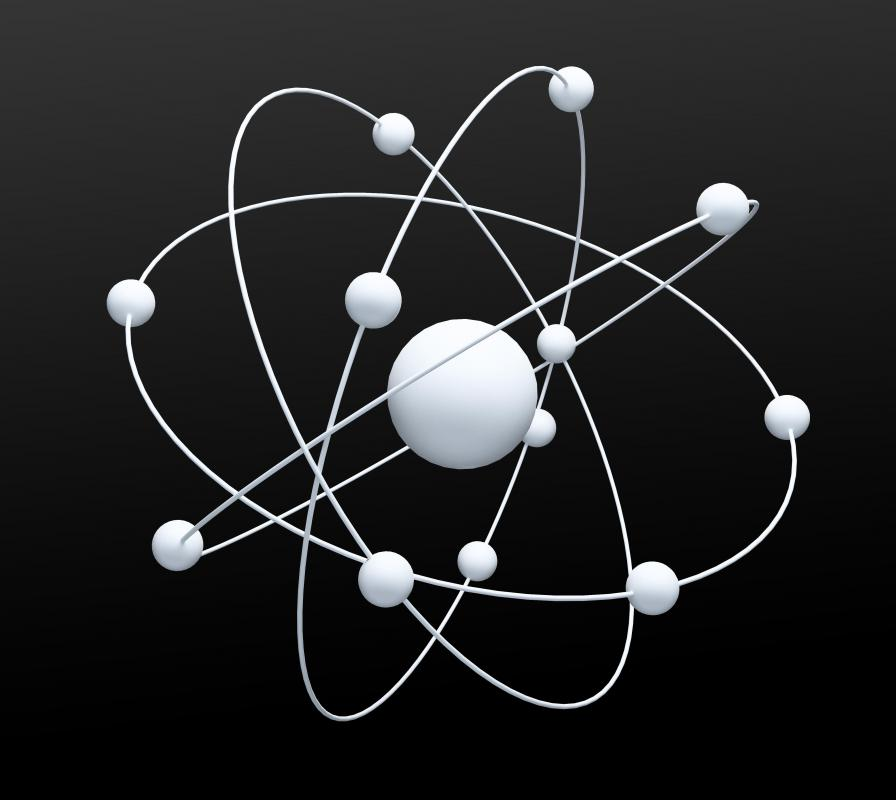# What is a Reducing Agent?

Danielle DeLee

A reducing agent is a term in chemistry that refers to an atom which donates electrons in an oxidation-reduction reaction. The atom that gains those electrons is said to be reduced. The reduced atom is called the oxidizing agent; it takes electrons from the oxidized atom, which is another name for the reducing agent.Whether an atom acts as an oxidizing agent or a reducing agent depends on the direction in which the reaction is spontaneous.

If an electron leaves an atom it must go somewhere else, so the processes of oxidation and reduction go hand in hand. Together, they form a class of reactions called oxidation-reduction reactions, also known as redox reactions. These reactions generate a flow of electrons, so they have electrical potential.

Scientists can harness the potential of oxidation-reduction reactions to create electricity. This is the concept behind the potato battery, a common science experiment. The experimenter puts one zinc lead and one copper lead into the potato. The free-floating ions in the potato facilitate the electron flow between the two leads by preventing a buildup of positive charge around the leads that would stop the reaction. Electrons flow from the lead that acts as a reducing agent to the lead that acts as an oxidizing agent; in the process, atoms from the reducing lead enter the solution of the potato, while ions surrounding the oxidizing lead are converted into metal on the surface of the original lead.

If an atom is an oxidizing agent in a reaction, it would be a reducing agent if the reaction were reversed. Whether an atom acts as an oxidizing agent or a reducing agent depends on the direction in which the reaction is spontaneous. Reactions occur spontaneously if their products are relatively more stable than their reagents. Scientists can predict the spontaneity of oxidation-reduction reactions based on their electric potential.

To evaluate a potential oxidation-reduction reaction, scientists first break the reaction into half reactions, which represent the loss of electrons, or reduction. In the case of the potato, zinc and copper can both form ions with a charge of positive 2. Thus, the half reactions are Zn+2 + 2e- --> Zn and Cu+2 + 2e- --> Cu.

The next step is to find the direction of electron flow. The experimenter does this using a table of standard reduction potentials, which gives a potential for each half reaction. If the direction of the half reaction is reversed, its potential has the same magnitude, but its sign changes. The potential for the half reaction of zinc is -0.76 volts, while that of copper is 0.34 volts.

This means that zinc is a stronger reducing agent than copper, so in this reaction zinc acts as the reducing agent. The overall reaction in the potato battery is Zn + Cu+2 --> Zn+2 + Cu, which generates 1.10 volts of electricity in the wire connecting the leads. If the zinc lead were replaced with a silver lead, however, then copper would be the reducing agent, since the half reaction of silver, Ag+ + e-, has a standard reduction potential of 0.80 volts. The battery would generate 0.46 volts.

## You might also Like